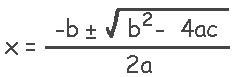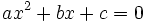Article Summary: The quadratic equation has many practical applications in the world beyond school. You may not think you need to know it now; however the higher paying jobs go to those who can use the quadratic equation to design safe and useful products for people.

In reality the quadratic equation as many functions in the scientific and mathematical world. The equation is used to find shapes, circles, ellipses, parabolas, and more. The quadratic formula looks a little menacing, however it is not .The quadratic equation is different from the formula and looks like this:and we will be discussing the quadratic equation.

The quadratic equation is used to find the curve on a Cartesian grid. It is primarily used to find the curve that objects take when they fly through the air. For example a softball, tennis ball, football, baseball, soccer ball, basketball, etc. It also used to design any object that has curves and any specific curved shape needed for a project. The military uses the quadratic equation when they want to predict where artillery shells hit the earth or target when fired from cannons. So if your goal is to go into the military and work with artillery or tanks, you will be using the quadratic equation on a daily basis.

Other uses of the quadratic equation include explaining how planets in our solar system revolve around the sun. Our planets were initially tracked by early scientists, who did not have the advantage of computers, and they used the quadratic equation to determine how planets in our solar system do not have circular orbits - they have elliptical orbits.

Newton also based his laws of motion on the quadratic equation by defining the acceleration of objects and forces that act upon them. He based his laws on objects falling and moving, taking into consideration the objects are on a spinning object (earth), which is orbiting the our sun. Newton was not aware of the forces that act on our solar system from the rotation of the Milky Way Galaxy. Do you think this would have mad a difference in his calculations? No the answer would still be the same; however it would have taken him longer to calculate.

The quadratic equation is used by car makers to determine how much and what type of brakes are needed to stop a car going at various speeds, while it is still on the drawing boards. This and other design functions which use the quadratic equation are part of the design steps of a new car, truck, motorcycle, and other types of automobiles.

When a police officer investigates a car accident scene, he/she uses the quadratic equation in their efforts to determine what velocities the cars when traveling when the collision occurred. Also, who was at fault and why the vehicles were damaged the way they were. These calculations are also used by car designers to develop an even safer care for occupants during future collisions.

The quadratic equation is used in the design of almost every product in stores today. The equation is used to determine how safe products are and the life expectancy of products, such as when they can expect to quit working. Designers can then see what needs to be changed in the product to make it last longer.

Another area that the quadratic equation is helpful is in the design of sound systems, such as: speakers and electronic circuits for vibrating the speakers. Speakers send out sound waves and these sound waves vibrate or resonate, sometimes causing unwanted cancellations of sound waves. Designers use this equation to redesign the circuits and speakers so sound waves reinforce each other and do not cancel each other out for the best sound quality.

The quadratic equation has many practical applications in the world beyond school. You may not think you need to know it now; however the higher paying jobs go to those who can use the quadratic equation to design safe and useful products for people.